# Arrival Rate Bias in A/B Testing

This is the second post in our two-part series on arrival rate bias correction in A/B Testing. I am grateful to my Wealthfront Data Science colleagues Hsiu-Chi Lin and Daniel McAuley for their invaluable input. Part 1 may be found here.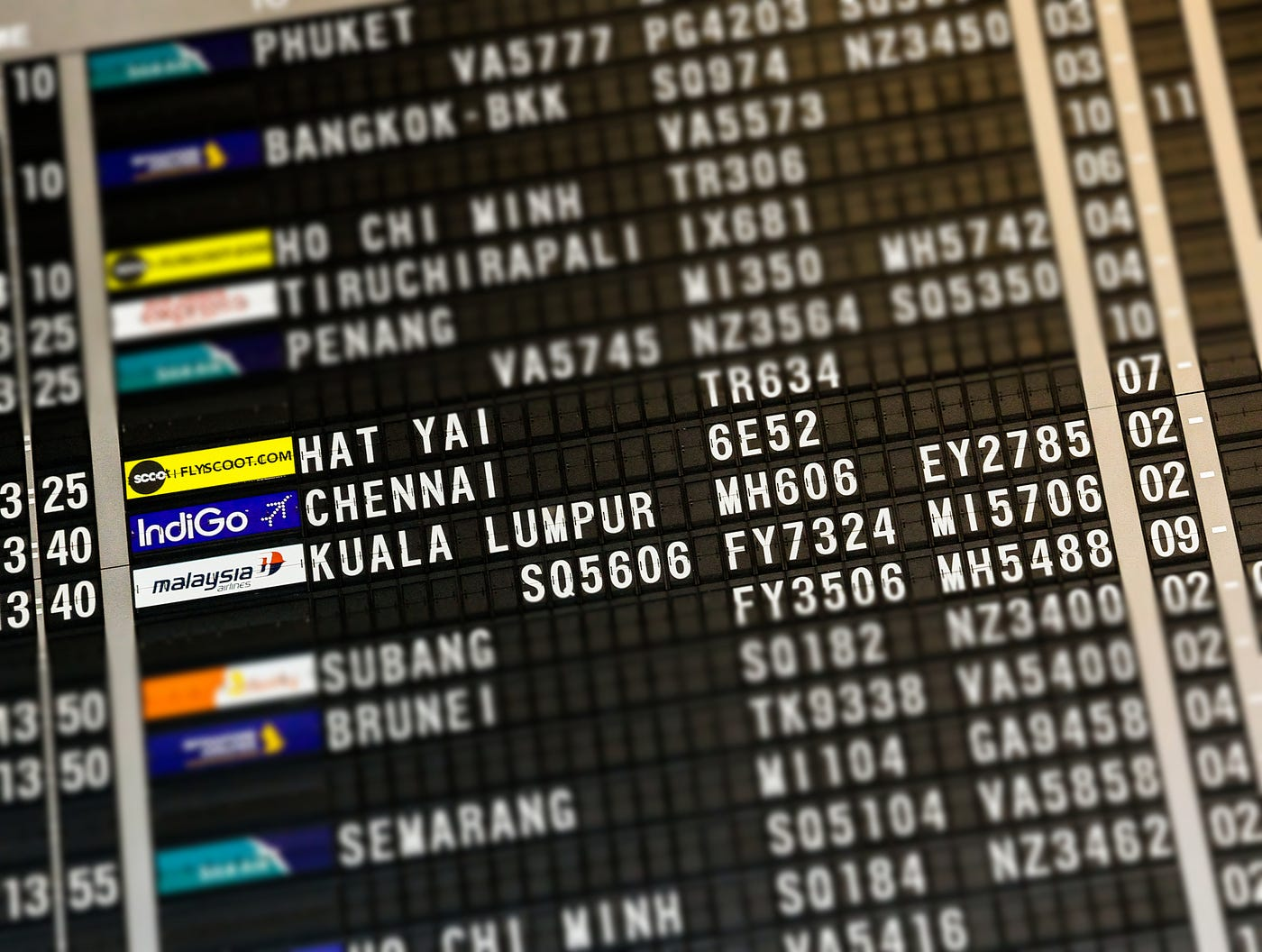“flight board highlighting Hat Yai, Chennai, and Kuala Lumpur” by Benjamin Wong on Unsplash

In our previous post, we introduced the problem of “Arrival Rate Bias”, a selection bias caused by heterogeneity in both rates of arrival and response to treatment in certain kinds of A/B testing frameworks. In particular, this is a problem when samples join an experiment — or are “bucketed”, using our terminology — upon arrival to a landing page. We proposed inverse propensity weighting (IPW) as a method to obtain an unbiased estimate of the average treatment effect (ATE), given our “first-come, first-served” bucketing mechanism. In our view, this solution is attractive because it can be adapted to our real-time monitoring setup and can, in principle, capture unobserved factors that regression cannot. In this second post, we develop this solution and investigate its properties in a set of simulations.

As before, we define K as the ex-ante number of clients that are eligible to be bucketed into an experiment; N as the number that have been bucketed so far; and R as the ratio N / K. It is important to understand that N and R increase as more samples arrive, until the desired number of samples N* = R*K are collected.

We explained that for a given value of R, the i’th sample (a client with arrival rate λ_i) should be given the weight

where the denominator is the client’s ex-ante “propensity” to be bucketed. Under our bucketing mechanism, this is the probability that the client would have been among the first N = RK clients to arrive to the relevant landing page. (If R = 1, w_i = 1 for all i because every client is bucketed with probability 1.) The IPW estimator for a given N is then the weighted difference in means

where Y is the response variable being measured, I_v and I_c are the sets of clients in the treatment (“variant”) and control groups that have been bucketed, and n_v = |I_v| and n_c = |I_c|

# Estimating the Propensity

A lingering question is how to compute P(bucketed | λ_i; R). In our previous post, we outlined a simple hierarchical model in which λ has a lognormal distribution and the arrivals for each client follow a Poisson process. This permits numerical computation of the propensity by integration.² However, these are fairly strong assumptions that might not hold up to a closer examination of the data. A second approach that we adopt is to estimate the propensities empirically using data on clients’ past arrival patterns, the key assumption being that these patterns are relatively stable through time.

A simple method is to choose some arbitrary reference time T, compute arrival rates using arrival history before T, and look at the ordered arrivals after T. The chart below shows the empirical propensity curves for different values of R. A given curve depicts the proportion of clients with arrival rates in percentile x that were among the first fraction R to arrive after T. When R = 1, the curve should be a horizontal line at y = 1.

One of the reasons that IPW works in practice is that some clients who are ex-ante unlikely to be bucketed happen to arrive by chance. For small values of R, however, some clients who are very unlikely to be bucketed may arrive anyway! They will be given very high weights and therefore vastly inflate the variance of our IPW estimator. A data scientist or product manager who is tracking one of our experiments in real time might then see large jumps in the estimated ATE early in the experiment. This is undesirable, so we suggest a couple of tweaks to the propensity-weighting algorithm below to encourage more desirable small-R behavior.

## Arrival Rate Binning

One tweak is to compute propensities over binned arrival rates. Technically this is already done to estimate the empirical propensities as described above, but it makes sense to coarsen the bins from percentiles to deciles or even quintiles. The “binned weight” for each λ_i is computed as

where B(λ_i) is the set of λ_j in the bin to which λ_i belongs. Even for relatively small values of R, the probability of arrival by a client in the lowest decile is not exceedingly small, so the bin weight will not be too large, as shown below. In our simulations, this helps to stabilize the variance of our estimator at the price of a small (tolerable) amount of bias.

## Propensity Averaging

Choosing a single reference time T is likely to lead to empirical propensities that are highly idiosyncratic to the arrival patterns around that particular time. As a simple example, two clients that visit Wealthfront with the same daily frequency but at different times of day will have different estimated arrival rates using the estimator that we proposed in our previous post.³ To improve this, we choose a number of reference times uniformly at random within some predefined time window and average, within each bin, the propensities computed with each. Plotting the log of the average weight over bins (an average of averages) shows the resulting reduction in the variance of the weights, compared to each of the individual reference times (red curves). Notice that the greatest stabilization occurs when R is small; the weights must converge to 1 (0 for the logged weights) as R approaches 1.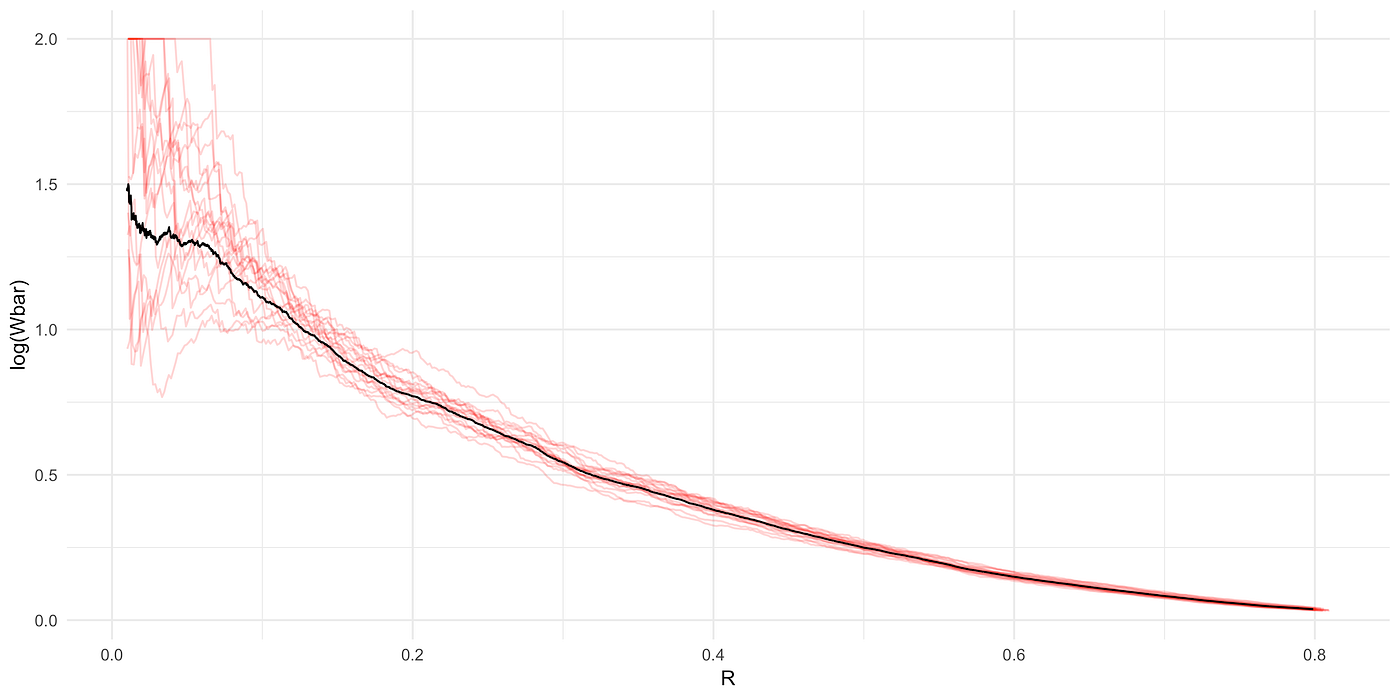Average bin weights (Wbar) computed with 20 reference times individually (red) and from the average of the propensities associated with each (black)

In A/B testing it is standard to choose the sample size N for an experiment that will yield some target level (often 80 percent) of statistical power, given a postulated effect size 𝜇 and the decision rule that rejects the null hypothesis (no effect) if p < 𝛼 (typically .05). This means that if the “true” effect is 𝜇, we will detect it with 80 percent probability. For a comparison of means, this amounts to stopping the experiment when the variance of the difference-in-means estimator \bar{𝜏} := \bar{Y}_v – \bar{Y}_c becomes smaller than a threshold value:

where 𝛽 = 1 – power. When the samples are weighted by w_i, the IPW estimator

will have higher variance than \bar{𝜏}, necessitating a larger sample size before the variance reaches the same threshold value as above. If the weights were fixed, it would be straightforward to make this adjustment. But there is an interesting dynamic at play: As more samples are bucketed into the experiment and R increases, the distribution of arrival rates in the bucketed sample becomes less biased relative to the population distribution, and the weights shrink toward 1. This means we actually need fewer additional samples than if we were simply sampling from the same biased distribution. Recognizing this dynamic is critical to understanding the following argument, which leads us to an implicit equation which can be solved numerically to find the bias-adjusted sample size N’. (The math below is for completeness; feel free to skip ahead to the section on our simulations.)

Let Y_v and Y_c be binary random variables — for the treatment (“variant”) group v and the control group c — derived from underlying functions p_v and p_c which are heterogeneous in the arrival rate λ, with conditional distributions

Also, define 𝜇_v = E_F(Y_i,v) and 𝜇_c = E_F(Y_i,c), where F is the “population” distribution of arrival rates among our K eligible clients, and the conditional average treatment effect

Then the variance of the IPW estimator above, for a given N = n_v + n_c with an implied arrival-biased distribution H(R), is

where in the third step we burn one w_i to transform an expectation over H into an expectation over F. (To see this, it helps to recall that w is also the likelihood ratio f / h, as detailed in our previous post.) At the threshold, the variance of the vanilla difference-in-means estimator is merely Var(\bar{𝜏}) = 2 * 𝜎²_𝜏 / N, where

Equating these and dividing out K gives the implicit equation

where R’ = N’ / K.

The exact form of the above will vary depending on the shape of p_v(λ) and p_c(λ) and the manner in which the propensities are estimated — some variations of which we discussed above — but the principle of equating variances is applicable in each case. Of course, p_v(λ) and p_c(λ) are unknown in advance of an experiment, but one of our findings is that for a range of a priori feasible functions, the adjusted sample size N’ depends much more strongly on the ATE 𝜇 and the variance 𝜎²_𝜏, the key inputs in the conventional power calculation. If we make the assumption of no heterogeneity in p_v and p_c, then the equal-variance condition above becomes

where \bar{W}_bin(R’) is the average of the binned weights at R’.⁴

# Simulations

Taken with the modifications we’ve proposed, the propensity weighting method seems to have mathematical properties that will help correct the arrival bias problem. But how can we have greater confidence that this method will be an effective antidote to our problem? And how do we convince other stakeholders in our A/B testing framework? To stress-test this method, we ran a series of simulations to give a coarse sense of how we should expect it to work in practice (and to check that our math is correct!).⁵ We maintain the assumption that each client’s arrivals follow a Poisson process and proceed as described thus far.

## Checking the Power Calculation

The first simulation is simply a sanity check that our adjusted sample size calculation is correct. Suppose that p_v(λ) = .34 and p_c(λ) = .3 (both constant) and λ ~ LogNormal(-3.8, .9). We can first estimate the propensity weights (with arrival rate binning and propensity averaging) by repeatedly — say, 100 times — drawing 10,000 λ_i and, for each i, generating X_i ~ Exponential(λ_i) for the first arrival time and Z_1i, Z_2i ~ Exponential(λ_i) to compute the estimate of the arrival rate \hat{λ_i} = 1 / (Z_1i + Z_2i). Ranking these samples by X_i and binning \hat{λ_i} into deciles allows us to compute the empirical propensities — the fraction of the 1,000 samples in each bin that are among those with the N earliest arrivals, for N = 1, …, 10,000. Averaging the bin propensities over 100 repetitions and then taking the average of weights over the bins gives \bar{W}_bin(R) for values of R between 0 and 1 at a resolution of .0001.

Now suppose that K = 50,000. With a target power of 60%, type I error of 5%, and the values of 𝜇 and 𝜎²_𝜏 implied by p_v and p_c, the standard power calculation for a two-sided t-test says that we need N = 2,661 samples, and the bias-adjusted calculation says that we need N’ = 4,646. To validate this, we run 600 iterations of the following:

1. Construct the arrival-biased distribution of λ by drawing K samples of λ_i from LogNormal(-3.8, .9), generating X_i | λ_i for each and estimating and binning \hat{λ_i} in the same manner as above, and keeping the samples with the N’ smallest values of X_i.
2. For each i in this filtered sample, make a random assignment to the treatment or control group, and draw either Y_i,v ~ Bernoulli(p_v(λ_i)) or Y_i,c ~ Bernoulli(p_c(λ_i)) according to the assignment.
3. Get the weight w_i for each sample by matching to the corresponding bin in the weight table at R’ = N’ / K.
4. Compute the IPW estimate \bar{𝜏}_w and its standard error⁶, and use this information to decide whether to reject the null hypothesis H_0 at the 95% significance level.

Close to 60% of the iterations result in rejection of H_0, suggesting that we successfully achieved our target power in this example.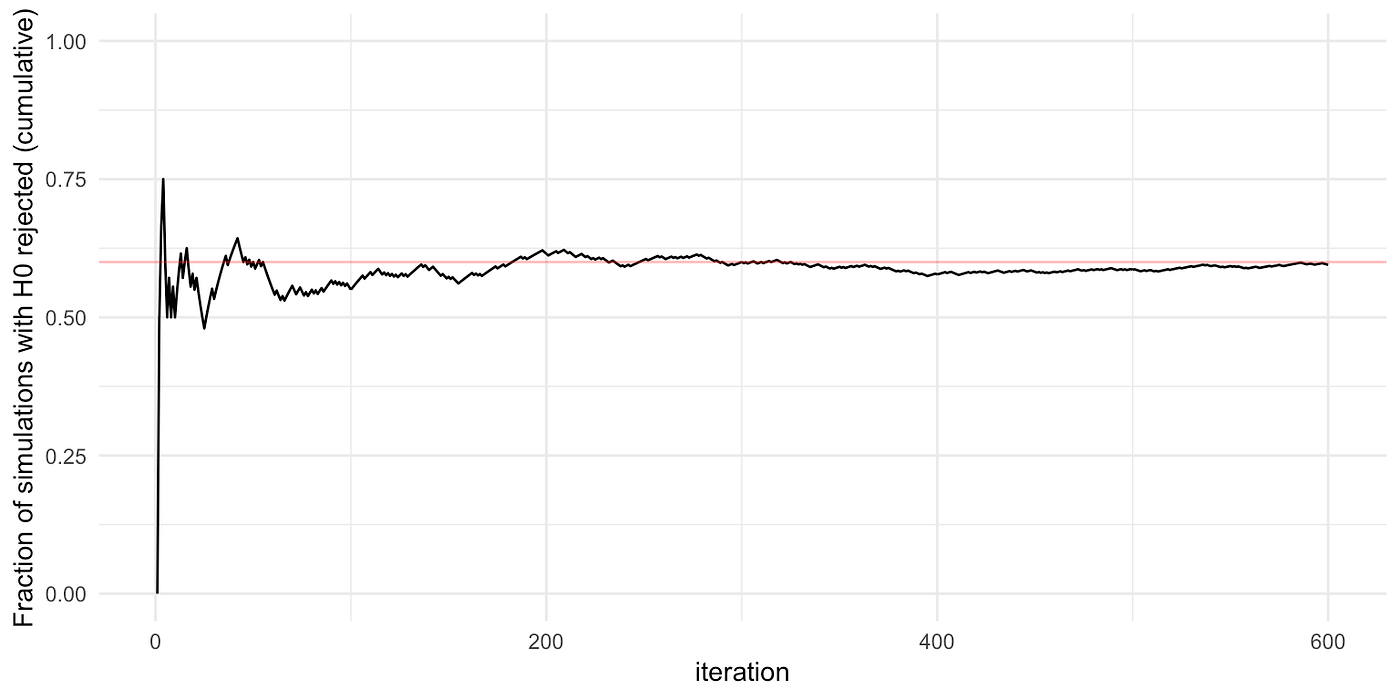The cumulative fraction of simulations in which the null is correctly rejected approaches the desired target power (60%).

## Simulating Time Series of the Estimated ATE

To get a sense of the effect of the severity of heterogeneity on the required sample size, we choose different functions 𝜏(λ_i) for the conditional ATE, from which the pair of functions (p_v(λ), p_c(λ)) defined above can be constructed.⁷ For simplicity, we consider functions which imply constant conditional variance 𝜎². (The steps are mostly similar to those in the previous section, but we present them again as pseudocode for readers that prefer this more structured format.) For each pair (𝜏(λ_i), 𝜎²):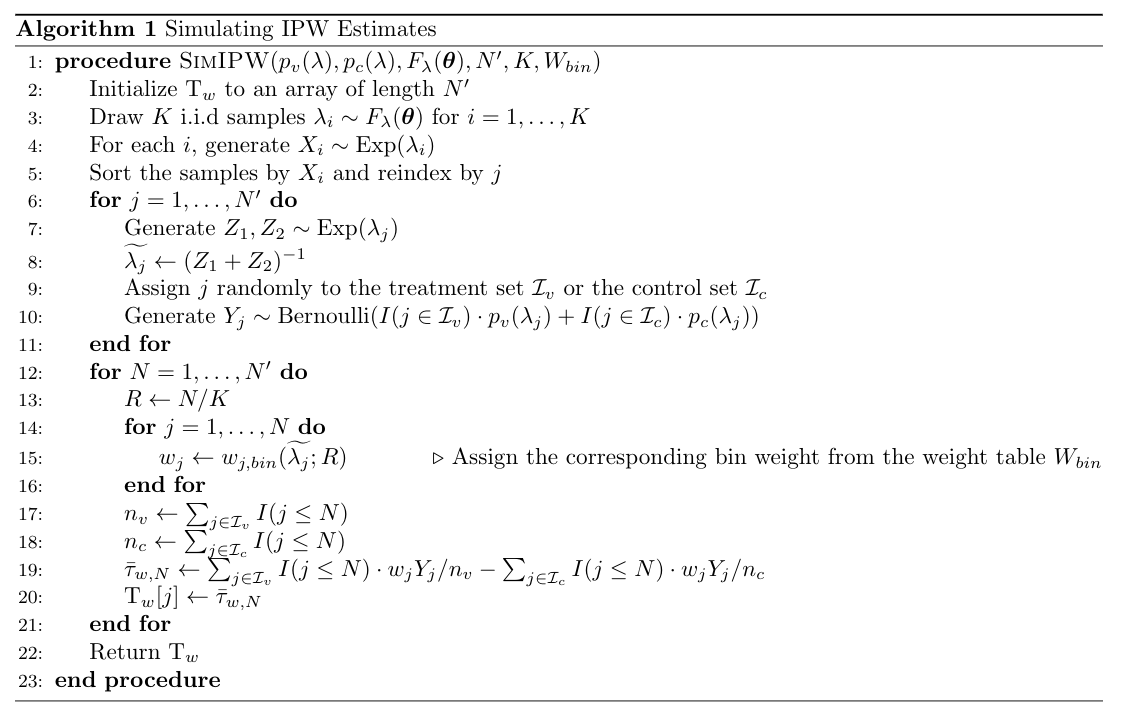A procedure to generate a time series of the ATE when samples arrive sequentially

The result of this procedure is a “time series” of the estimated ATE similar to what we might observe in our real-time A/B testing dashboard. In step 7(c), we can simultaneously compute the regular difference-in-means estimator to compare the series that we would see without any correction for arrival rate bias.

Below is one such simulated ATE time series, facetted by day (corresponding the arrival times X) and by the number of samples collected. The underlying simulated data are the same. The dashed line represents the “true” effect size and the colored series represent the estimated ATEs with 95% confidence intervals. The solid portion of the lines correspond to the sample collection period (terminating at the number of samples determined by the respective power calculations), and the dotted portions are the extension of the series that we would have seen had we collected additional samples.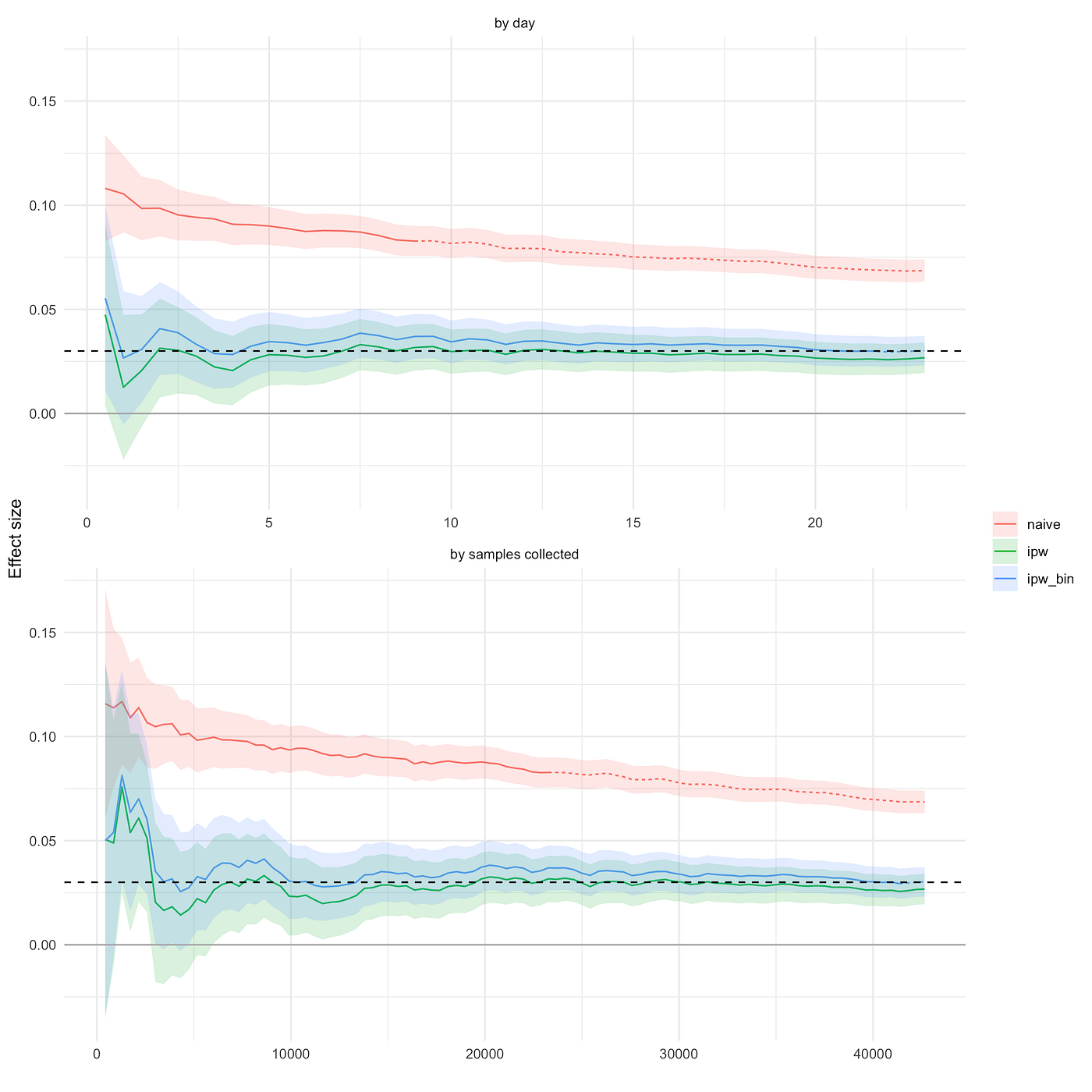Simulated estimates of ATE for 𝜇 = .03, 𝜎² = .2, 𝜏(λ) = -.7 + λ^(.0818), K = 200,000

The most striking observation is that the confidence intervals for the propensity-weighted estimates cover the true effect size for the entire duration of sample collection, while the naive estimate never does. (Though the latter must converge to the dashed line as N approaches K.) This is the key property that we need for real-time monitoring.

Looking at the solid portions of the series, we see that applying the propensity-weighted correction demanded roughly 2X the number of samples before the confidence interval of the estimate became sufficiently small. In this case, the series estimated with the binned weights (blue) runs a little bit higher than the series estimated without binning (green), perhaps reflecting the small amount of bias that we are willing to tolerate. In other simulations, the binned computation more clearly results in smaller deviations from 𝜇 early on.

(Note: In a real experiment, we will not know the true effect, so for the simulation we set the postulated effect size to .01 in the sample size calculation, resulting in more samples than necessary to achieve our Type I / Type II error targets. If we instead set this to .03 (or set 𝜇 = .01), we should expect to see 80% power in repeated simulations with the appropriate sample size, as in the previous subsection.)

We can also run different variations of this procedure, such as assuming p_v and p_c to be constant in our calculation of the adjusted sample size, and measuring the loss of power when p_v(λ) and p_c(λ) are not constant to varying extent.

# Conclusion

The results of our simulations give us confidence that inverse propensity weighting is, in principle, a feasible correction to arrival rate bias, in terms of both ease of implementation and accuracy of the resulting estimates. In practice, remaining challenges will likely arise and demand further corrections. One aforementioned example is that our solution relies heavily on the conceptualization and estimation of a client’s underlying Poisson arrival rate. In reality, clients may arrive to Wealthfront with more or less regularity; some may reliably log in at 8 AM every weekday, while others may log in in response to emails and product announcements on our side.

As we continue to run A/B experiments to learn about our clients’ preferences and behaviors, we will continue improving our underlying testing framework to get more accurate estimates of the effects of new products and features. As we’ve demonstrated here, a little bit of statistical thinking goes a long way toward making this a reality.

## Footnotes

¹ Another version of this estimator is one with weight normalization, in which n_v and n_c are replaced by the sums of the weights in the respective groups. See this chapter on importance sampling for a more detailed discussion on weight normalization and the notion of “effective sample size”.

² When λ follows a gamma distribution, the arrival-biased distribution (and therefore the propensity) has a closed form.

³ Recall that we proposed the inverse of the time since the penultimate session prior to T, which is unbiased estimator of the Poisson rate.

⁴ Note that if we somehow knew that no heterogeneity were present, then there would be no arrival rate bias to correct for! This exercise is meant to suggest a baseline for adjusted sample size conditional on using the IPW estimator to correct for an unknown degree of heterogeneity.

⁵ R code to replicate the simulations is available here. (soon)

⁶ The standard error is \sqrt{𝜎²_v / n_v + 𝜎²_c / n_c}, where 𝜎²_v = mean(w_i² * y_i) – mean(w_i * y_i)² for i in the variant, and likewise for 𝜎²_c.

⁷ For a conditional variance function 𝜎²(λ) := Var[Y_i,v – Y_i,c | λ_i], we can set p_v(λ) = (1 + 𝜏(λ) – \sqrt{1 – 2𝜎²(λ) – 𝜏(λ)²}) / 2 and p_c(λ) = p_v(λ) – 𝜏(λ). For constant conditional variance 𝜎²(λ) = 𝜎² we need 𝜎² ≤ (1 –max(𝜏(λ)²)) / 2.

--

--

--

## More from Allen Sirolly

data science @ wealthfront

Love podcasts or audiobooks? Learn on the go with our new app.

## How to Enter Your First Zindi Competition## Improving Analytics Engineering at mPharma — PART 1## Charted: The Covid Whiplash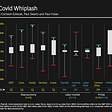## Draw a unique barplot using Matplotlib in Python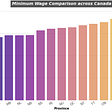## Covid-19 Outbreak Part 1 — More Than What Meets The Eye## Augmented and Virtual Reality Data Visualization## Measuring impact as an early career researcher (Part 1)## Update: How to Create Calendars in FileMaker with Monday Start of Week## Allen Sirolly

data science @ wealthfront

## Learning the Udacity A/B Testing Course through its Final Project (Part 1/3)## Telecom Churn Prediction — EDA## CLVTools: A powerful R package to evaluate your customers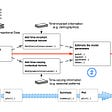## Causal Inference from Breast cancer Diagnosis